## Overview

The overarching goal of this project is to experiment with noise algorithms in order to generate realistic terrains. The name of this project is transparent in conveying its purpose - a terrain generator. The idea is the user of the application will be able to modify certain settings and generate randomized terrains in 3 dimensions. The libraries that support this application include OpenGL, GLFW, Eigen, tiny_obj_loader, and stb_image. The application is written in C++ and built using CMake.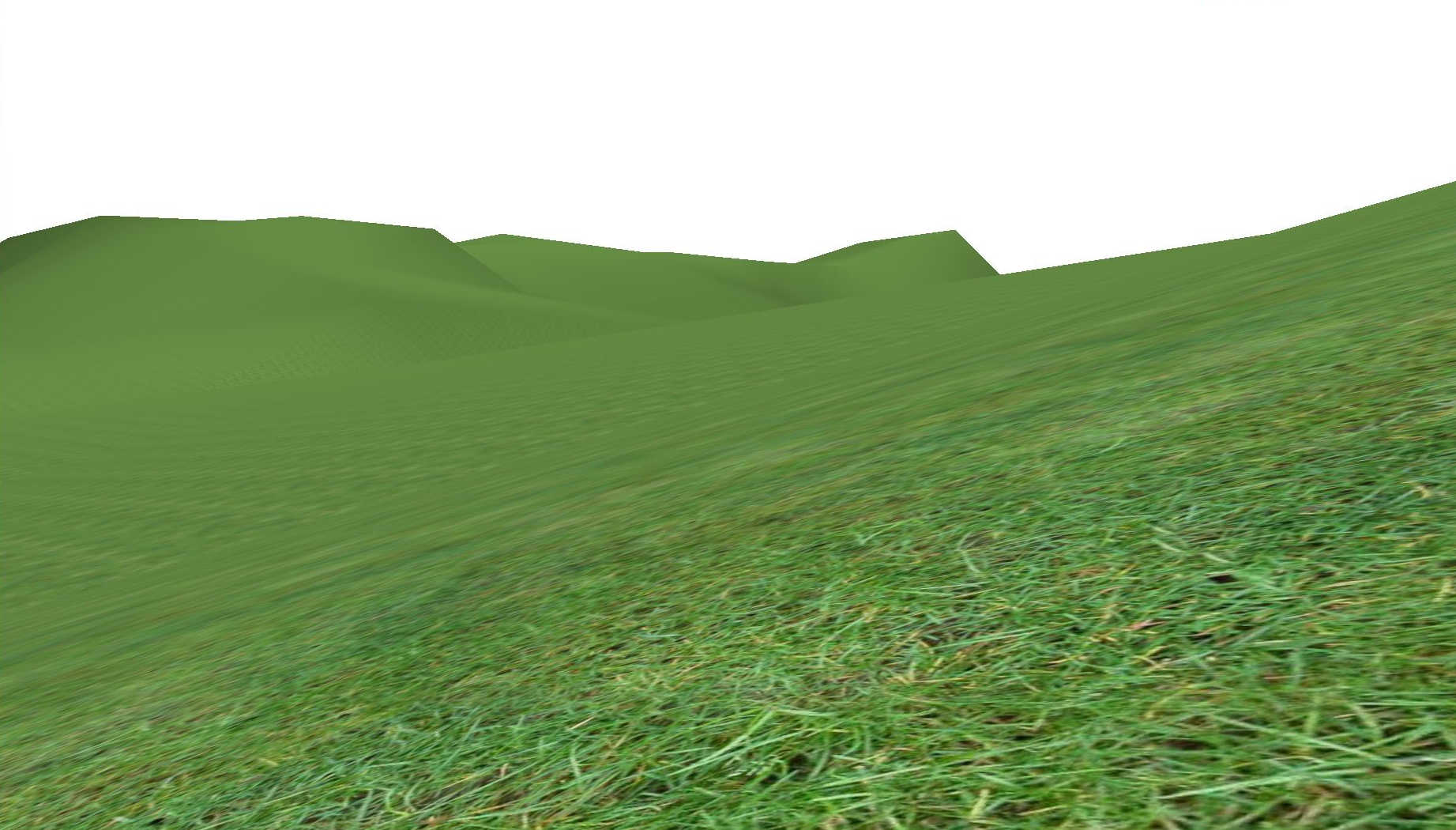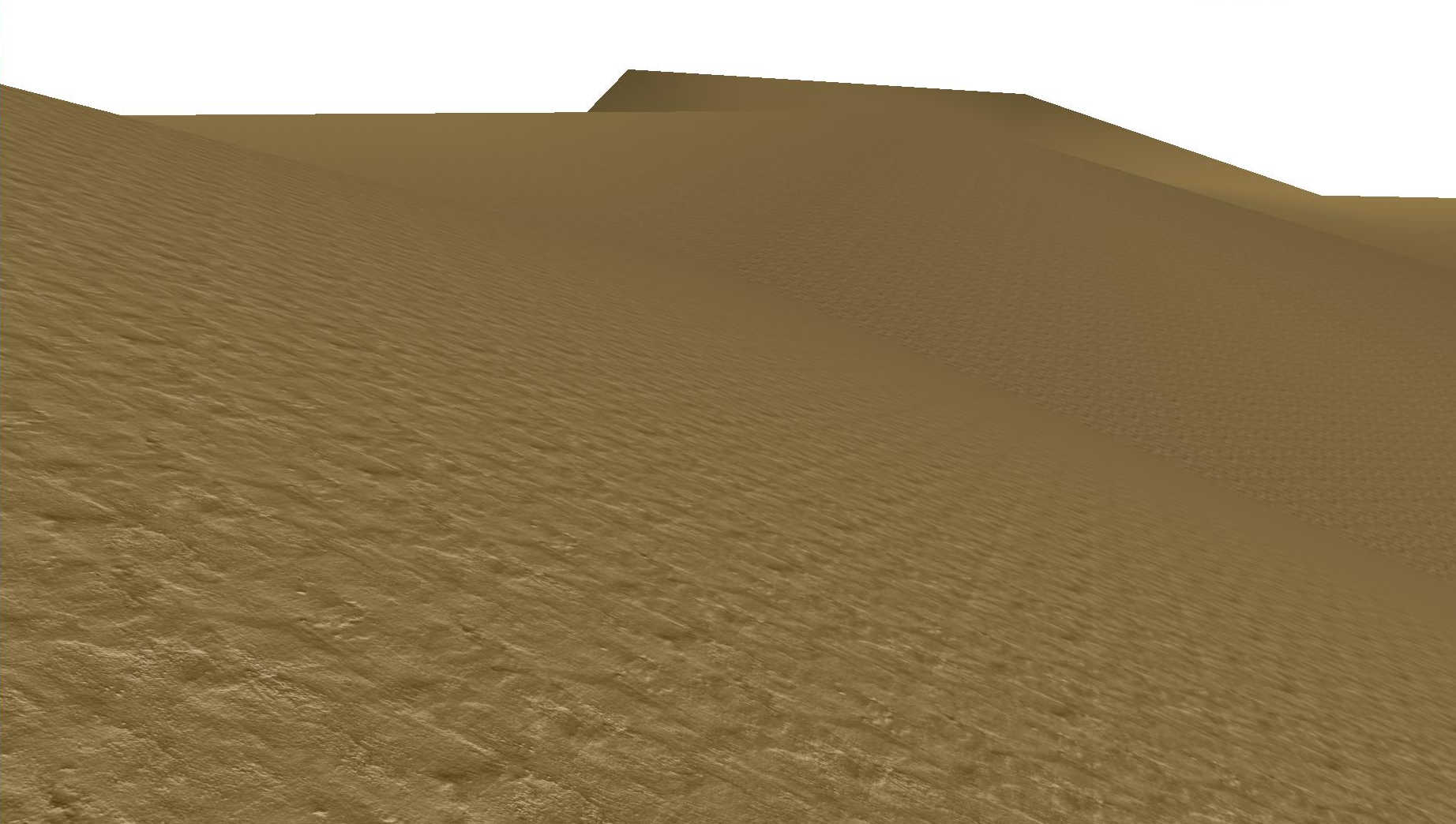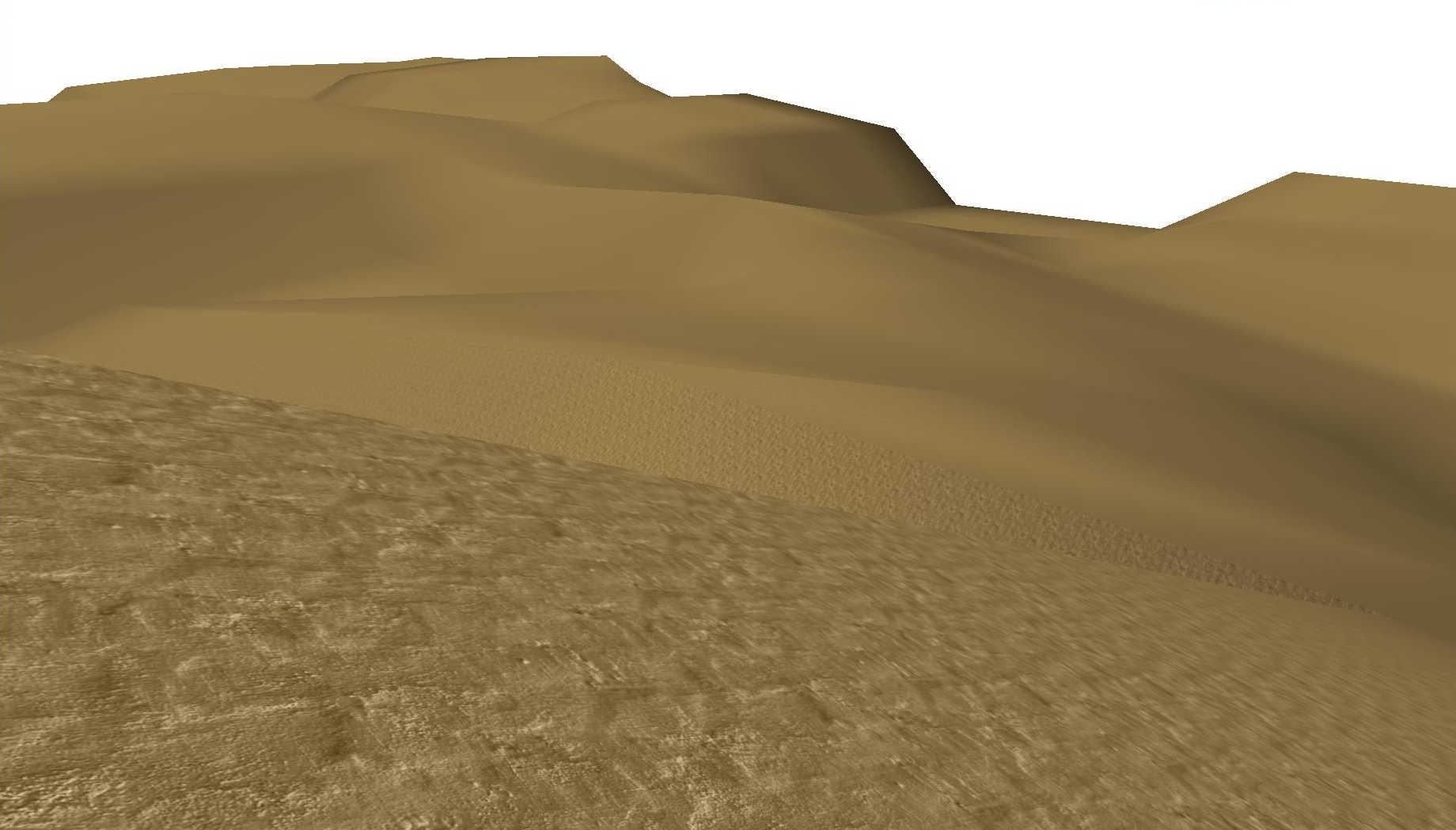The following is an itemization of the terrain settings the user is able to adjust:

Length Identifies the length and width of the square terrain.

Height Identifies the initial height variable used during the height computation. The actual height will vary depending on the octaves and persistence settings.

Grid Size Identifies the number of randomized points in the length and width dimensions of the square terrain. These points are equally spaced apart. The total number of grid points is thus the square of this variable. Increasing the grid size will intensify the randomness of the terrain.

Precision Specifies the number of linearly interpolated points in the length and width dimensions internal to each randomized grid point. Increasing the precision will exponentially inflate the triangle density and therefore the fineness or “precision” of the terrain.

Octaves Specifies the number of octaves for the noise algorithm. Higher octaves signifies rockiness while lower octaves signifies flatness of the terrain.

Persistence Specifies the persistence of the noise algorithm. Similar to octaves, higher persistence will lead to rockiness or randomness of adjacent positions of the terrain.

Biome Defines the type of environment the terrain inhabits. For the current progress so far, there are only two biomes: desert and grassland which are merely textures.

## Running Instructions

Once the project is unzipped, navigate to the build directory and execute the follow command by filling in the template:

cmake ..; make -j4; ./FinalProject

For example:

cmake ..; make -j4; ./FinalProject ../resources 700 150 10 3 4 0.5 1

If not enough arguments are provided, the application will fail to run and provide you the correct argument format. If too many arguments are provided, the excess arguments are ignored. If any input is not within the inclusive range emphasized above, that input is ignored and a default value is used instead.

The required libraries not provided include OpenGL, GLFW, and Eigen. Tiny_obj_loader and stb_image are provided in the src directory. If any file outside of the build directory is tampered with, the application is not guaranteed to run properly or at all.

## Algorithm Explanation

The terrain is a generated 3 dimensional interconnected set of rendered triangles. The x and z coordinates are determined by the length setting. The y coordinate, or the height, is computed using my implementation of a variation of the Perlin Noise algorithm from the article ​How to Use Perlin Noise in Your Games​. Essentially, the terrain is a height field with the height as perlin noise.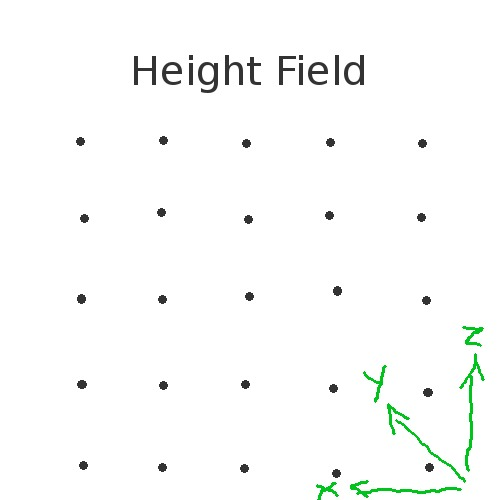First, I generate a 2 dimensional array of completely random noise. Then I use that noise array to generate a 2 dimensional smoothed noise array which has its noise values smoothed out or close to each adjacent noise. This smoothed noise array is used as the basis for the perlin noise algorithm.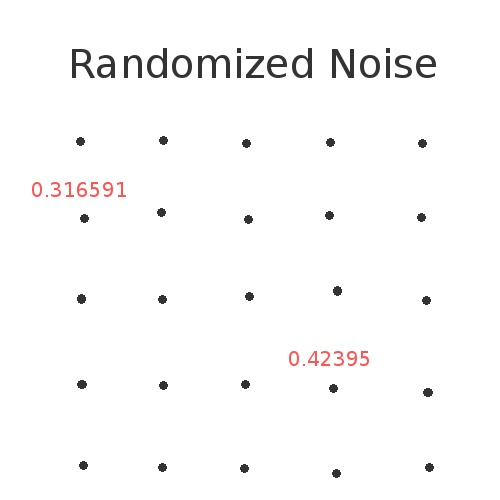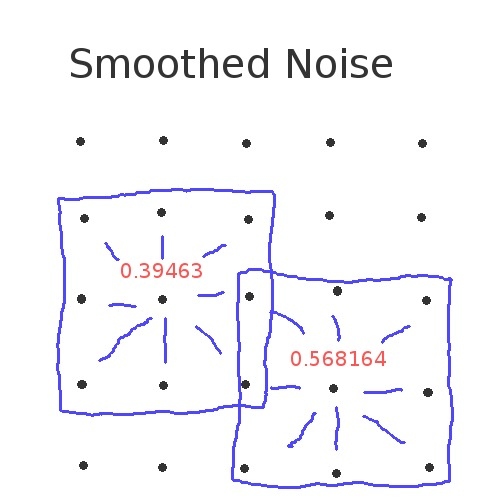Each octave has an amplitude and a wavelength which contributes to a total influence value which will be the height at the grid point x and z. The amplitude is computed using the persistence setting. For each successive octave, the amplitude is multiplied by the persistence. My algorithm sums each octave from the smooth noise 2 dimension array to ascertain a sum which is used as the height for that grid point. The diagram below illustrates this. The color would be substituted by the height.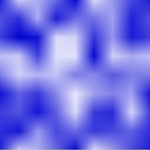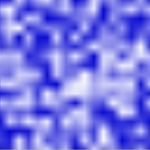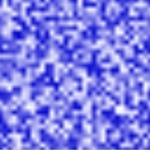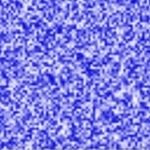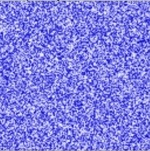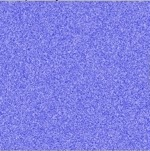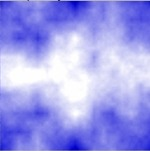Source: 

The positions are then calculated from the precision setting. The square of the precision describes the number of internal grids for every noise grid point.

Once the positions are generated, the normals are computed as the cross product of the dx and dz vectors which are the right minus left vector and forward minus backward vector respectively.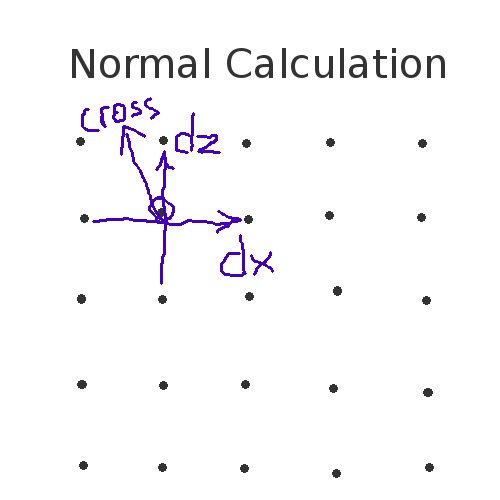Next, the texture coordinates are generated. The texture coordinates are applied linearly across the whole terrain, and then scaled by the length variable. This provides a repeating texture look which is sufficient for seamless textures.

Lastly, the indices are calculated and the terrain is rendered.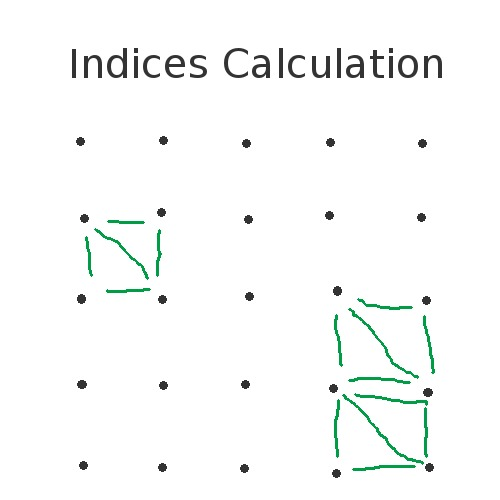## Technical Difficulties

Originally, I used a gradient noise implementation variation of Perlin Noise from . Then I redesigned it using the octave implementation because I found it easier to implement.

## References

 Helper classes provided by Professor Shinjiro Sueda